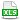HomeTechniques and Tips@RISK DistributionsF Distribution

# 3.37. F Distribution

Applies to: @RISK 5.0 and newer

Does @RISK have an F distribution?

Select Define Distributions » Continuous » F, or Insert Function » Continuous » RiskF.

With @RISK 5.x:

Before release 6.0, @RISK did not have an F distribution (Fisher-Snedecor distribution, variance ratio distribution) among the pre-programmed list. If you still have @RISK 5.x, you can easily create one yourself with a ratio of chi-squared distributions. According to Evans, Hastings, Peacock Statistical Distributions 3/e (Wiley, 2000), page 92:

The variate F:n,m is related to the independent Chi-squared variates χ²:ν and χ²:ω by

F:n,m ~ [(χ²:ν)/ν] / [(χ²:ω)/ω]"

Therefore, to get a distribution of F(A1,A2), you can program

=RiskMakeInput( (RiskChiSq(A1)/A1) / (RiskChiSq(A2)/A2) )

The attached workbook shows this, and requires @RISK 5.0 and later. (In earlier versions of @RISK, you can still do the calculation, but the RiskMakeInput wrapper isn't available. RiskMakeInput, which lets you treat a calculation as a distribution for most purposes, was new in @RISK 5.0.)

Last edited: 2015-06-19

•F_distribution.xls